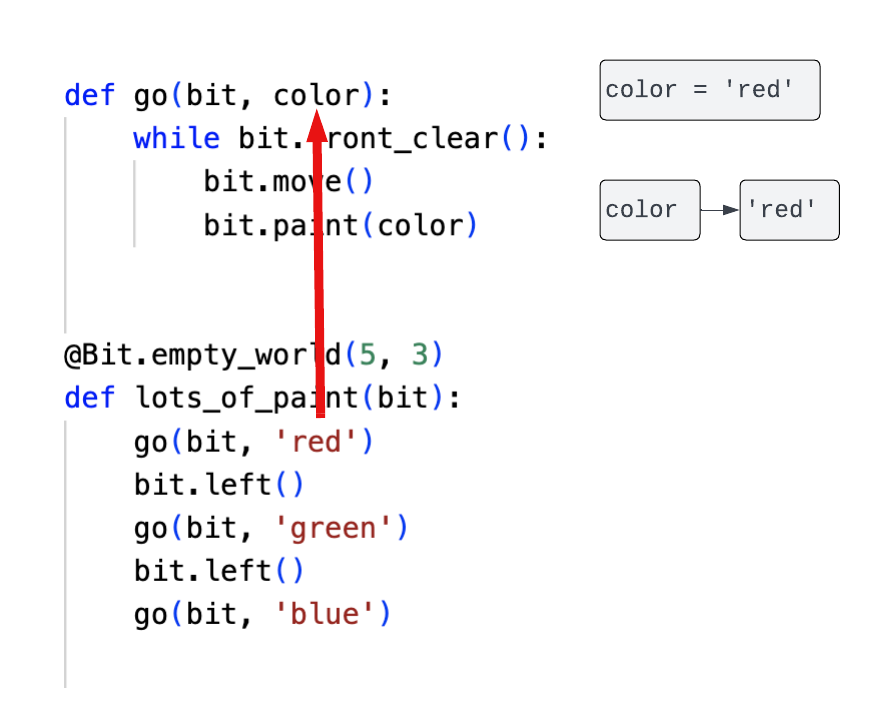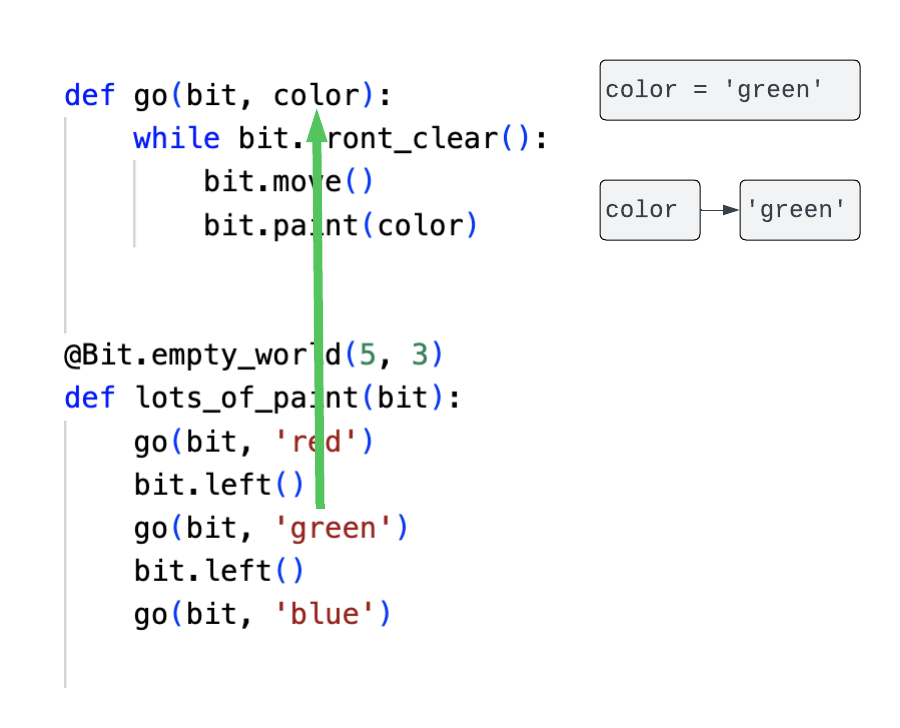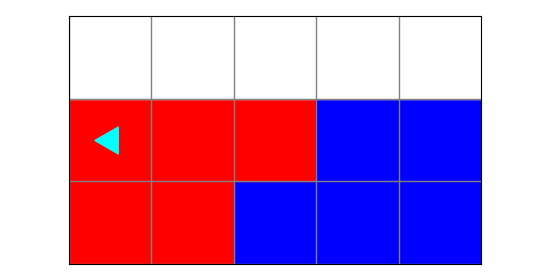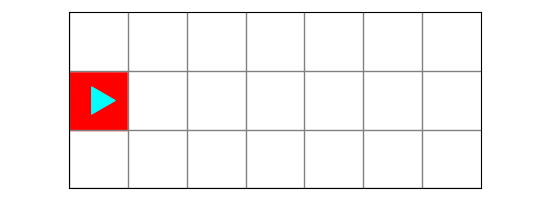Computer Science

# Variables

Consider the following code:

``````def paint_green(bit):
while bit.front_clear():
bit.move()
bit.paint('green')``````

This tells Bit to keep moving and painting green as long as the front is clear. So Bit will paint an entire row or column green, depending on which direction it is facing.

But what if we also want to paint rows and columns red? And yellow and green and brown and blue?

We would need to write a separate function for each one! Not great!

## Functions can have multiple parameters

Never fear, it turns out that functions can take multiple parameters:

``````def go(bit, color):
""" Given a bit and a color, move and paint that color until blocked """
while bit.front_clear():
bit.move()
bit.paint(color)``````

The `go()` function takes two parameters:

• a Bit
• a color

Inside the `go()` function, the `variable` called `bit` is equal to the Bit, and the variable called `color` is equal to whatever color you give it. You can see this in action using the file called `go_color.py` in the zip file above:

``````from byubit import Bit

def go(bit, color):
""" Given a bit and a color, move and paint that color until blocked """
while bit.front_clear():
bit.move()
bit.paint(color)

@Bit.empty_world(5, 3)
def lots_of_paint(bit):
""" Paint colors until blocked """
go(bit, 'red')
bit.left()
go(bit, 'green')
bit.left()
go(bit, 'blue')

if __name__ == '__main__':
lots_of_paint(Bit.new_bit)``````

Bit starts in an empty 5x3 world. It does the following:

• paints a row red
• turns left
• paints a row green
• turns left
• paints a row blue

The result is this world:## Parameters become variables in a function

To see how parameters to a function work, look at this diagram:The first time this code calls `go()`, it uses `'red'` as the second parameter. This means that inside the `go()` function, there is a variable called `color` that is equal to the string `'red'`.

The second time this code calls `go()`, we have this situation:This time, the code uses `'green'` as the second parameter. This means that inside the `go()` function, the variable called `color` is equal to the string `'green'`.

Notice that we use the notation `color → 'red'` and `color → 'green'` in this pictures. This indicates that a variable has a `name` (color) and it references a `value` (‘red’ or ‘green’). The value of a variable can change over time.

## Changing the value of a variable

You can change the value of a variable any time using this syntax:

``my_color = 'red'``

Here, the variable is called `my_color` and its value is `'red'`. Using our notation, `my_color → 'red'`.

Then you can call functions using this variable:

``````my_color = 'red'
go(bit, my_color)
my_color = 'blue'
go(bit, my_color)``````

The first time, `my_color → 'red'` and so inside `go()`, `color → 'red'`.The second time, the value of `my_color` changes, so now `my_color → 'blue'` and inside `go()`, `color → 'blue'`.## Painting with different colors

You can see this in action with the file called `color_variables.py` in the zip file above:

``````from byubit import Bit

def paint_two(bit, color):
bit.paint(color)
bit.move()
bit.paint(color)

def paint_three(bit, color):
paint_two(bit, color)
bit.move()
bit.paint(color)

@Bit.empty_world(5, 3)
def run(bit):
first_color = 'red'
second_color = 'blue'

paint_two(bit, first_color)
bit.move()
paint_three(bit, second_color)

bit.left()
bit.move()
bit.left()

paint_two(bit, second_color)
bit.move()
paint_three(bit, first_color)

if __name__ == '__main__':
run(Bit.new_bit)``````

Here we have a function called `paint_two(bit, color)` that takes two parameters, a `bit` and a `color`. It does this:

• paint the color
• move
• paint the color

We have another function called `paint_three(bit, color)` that takes the same two parameters. It does this:

• call `paint_two()`
• move
• paint the color

This allows it to paint three colors.

Finally, we have two variables: `first_color → 'red'` and `second_color → 'blue'`. We use these two variables when we call the functions, so that we can:

• paint two blue squares (using `first_color`)
• paint three red squares (using `second_color`)
• turn around and go to the next row up
• paint two red squares (using `second_color`)
• paint two blue squares (using `first_color`)

Run this code and see the result:## Getting the current color

Bit has a useful function called `get_color()` that lets you get the color of Bit’s current square so you can store it in a variable. For example:

``found_color = bit.get_color()``

Consider the following problem. Bit is in a world with one color:Bit’s job is to find out what color this is and then fill in the rest of the row with that same color:But Bit doesn’t know what color it is going to have in that first square. It might be blue:Look a the file called `fill_a_color.py` to see how to do this:

``````from byubit import Bit

def go(bit, color):
while bit.front_clear():
bit.move()
bit.paint(color)

@Bit.worlds('color1', 'color2')
def fill_a_color(bit):
found_color = bit.get_color()
go(bit, found_color)

if __name__ == '__main__':
fill_a_color(Bit.new_bit)``````

In `fill_a_color()`, Bit first gets the current color and stores it in a variable called `found_color`. It then uses `found_color` when it calls `go()`.

Run this code and use the buttons to see that it solves both worlds. In the first world, `bit.get_color()` returns `'red'`, and so `found_color → 'red'`. In the second world, `bit.get_color()` returns `'blue'`, and so `found_color → 'blue'`.